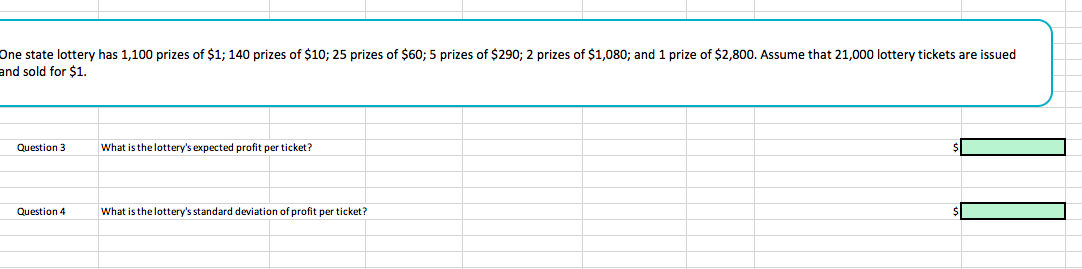# One state lottery has 1,100 prizes of \$1; 140 prizes of \$10; 25 prizes of \$60; 5 prizes of \$290; 2 prizes of \$1,080; and 1 prize of \$2,800. Assume that 21,000 lottery tickets are issuedand sold for \$1.Question 3What is the lottery's expected profit per ticket?Question 4What is the lottery's standard deviation of profit per ticket?

Question
134 viewshelp_outlineImage TranscriptioncloseOne state lottery has 1,100 prizes of \$1; 140 prizes of \$10; 25 prizes of \$60; 5 prizes of \$290; 2 prizes of \$1,080; and 1 prize of \$2,800. Assume that 21,000 lottery tickets are issued and sold for \$1. Question 3 What is the lottery's expected profit per ticket? Question 4 What is the lottery's standard deviation of profit per ticket? fullscreen
check_circle

Step 1

Given total lottery tickets sold 21,000 per \$1 each.

Number of \$1 prizes = 1100

Number of \$10 prizes = 140

Number of \$60 prizes = 25

Number of \$290 prizes = 5

Number of \$1080 prizes = 2

Number of \$2800 prizes = 1

Here the tickets are classified into ticket which won \$1,\$10, \$60, \$290, \$1080, \$2800 and won nothing. The number of tickets in each category is shown below in a table

Step 2

Profit obtained by selling \$1 prize lottery ticket = ticket prize - prize money = 1-1 = 0

Profit obtained by selling  \$10 prize lottery ticket = ticket prize - prize money = 1-10 = -9

Profit obtained by selling \$60  prize lottery ticket = ticket prize - prize money = 1-60 = -59

Profit obtained by selling \$290  prize lottery ticket = ticket prize - prize money = 1-290 = -289

Profit obtained by selling \$1080  prize lottery ticket = ticket prize - prize money = 1-10 80= -1079

Profit obtained by selling \$2800  prize lottery ticket = ticket prize - prize money = 1-2800 = -2799

Profit obtained by selling no  prize lottery ticket = ticket prize - prize money = 1-0 = 1.

Writing the table in terms of profit obtained per ticket and number of ticket sold given below

Step 3

The total tickets sold are 21000. So we calculate the probability of profit per each category will be total number of tickets sold/total number of tickets.

For example probability of \$0 profit per ticket  = total tickets with 1\$ prize/total tickets sold

=1100/...

### Want to see the full answer?

See Solution

#### Want to see this answer and more?

Solutions are written by subject experts who are available 24/7. Questions are typically answered within 1 hour.*

See Solution
*Response times may vary by subject and question.
Tagged in

### Statistics